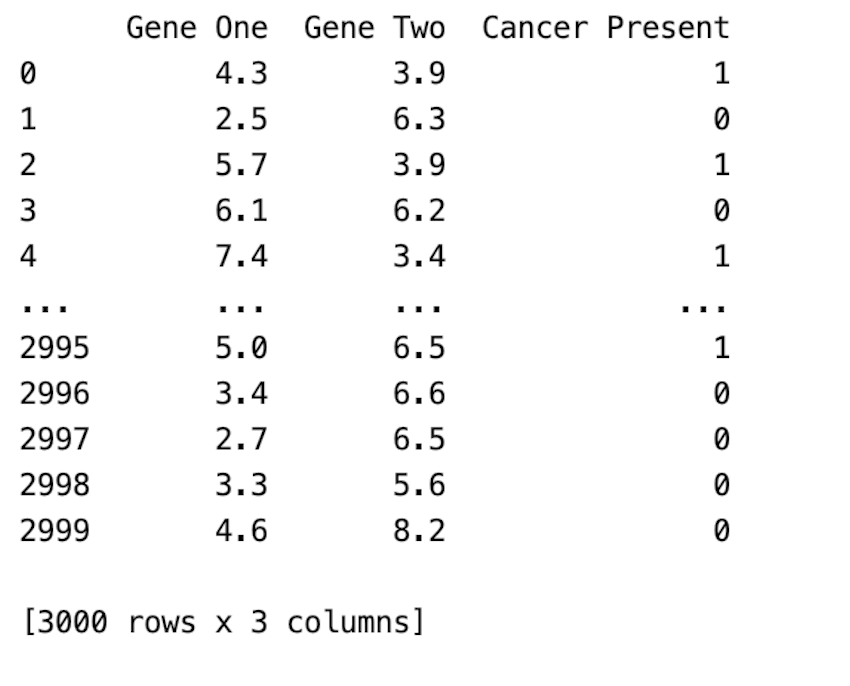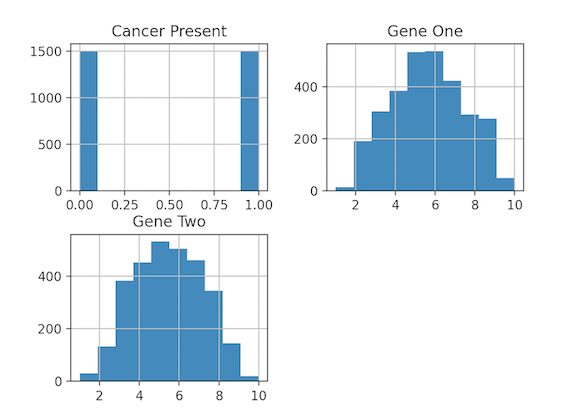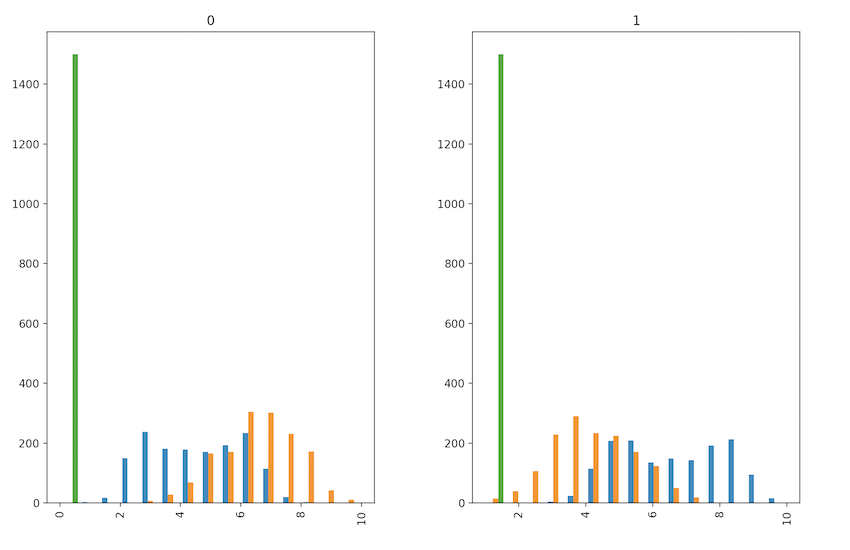# How to Create a Histogram from Pandas DataFrame?

• Last Updated : 19 Dec, 2021

A histogram is a graph that displays the frequency of values in a metric variable’s intervals. These intervals are referred to as “bins,” and they are all the same width.

We can create a histogram from the panda’s data frame using the df.hist() function.

Syntax:

DataFrame.hist(column=None, by=None, grid=True, xlabelsize=None, xrot=None, ylabelsize=None, yrot=None, ax=None, sharex=False, sharey=False, figsize=None, layout=None, bins=10, backend=None, legend=False, **kwargs)

### Example 1: Creating a basic histogram( histogram for individual columns)

We use df.hist() and plot.show() to display the Histogram.

CSV file used: gene_expression.csv

## Python3

 `# import libraries and packages ` `import` `numpy as np ` `import` `pandas as pd ` `import` `matplotlib.pyplot as plt ` `import` `seaborn as sns ` ` `  `# reading the CSV file ` `df ``=` `pd.read_csv(``'gene_expression.csv'``) ` ` `  `# displaying the DataFrame ` `print``(df) ` ` `  `# creating a basic histogram ` `df.hist() ` `plt.show() `

Output:### Example 2: Creating a modified histogram(plotting histogram by the group)

In this example, we add extra parameters to the hist method. We have changed the fig size, no of bins is specified as 15, and by parameter is given which ensures histograms for each cancer group are created.

## Python3

 `# import libraries and packages ` `import` `numpy as np ` `import` `pandas as pd ` `import` `matplotlib.pyplot as plt ` `import` `seaborn as sns ` ` `  `# reading the CSV file ` `df ``=` `pd.read_csv(``'gene_expression.csv'``) ` ` `  `# displaying the DataFrame ` `print``(df) ` ` `  `# creating a basic histogram ` `df.hist(by``=``'Cancer Present'``, figsize``=``[``12``, ``8``], bins``=``15``) ` `plt.show() `

Output:My Personal Notes arrow_drop_up
Recommended Articles
Page :